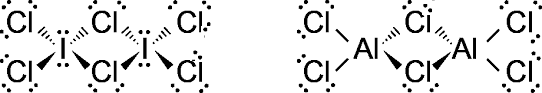Courses
Courses for Kids
Free study material
Free LIVE classes
MoreLIVE
Join Vedantu’s FREE Mastercalss

# Find out the similarities between ${ I }_{ 2 }{ Cl }_{ 6 }$ and ${ Al }_{ 2 }{ Cl }_{ 6 }$.A. both have ${ 3C-4e }^{ - }$ bond.B. both have ${ sp }^{ 3 }$ hybridization for the central atom.C. both are non-polar. D. all are correct.Verified
333.9k+ views
Hint: Electronic configuration of I = $\left[ Kr \right] { 4d }^{ 10 }{ 5s }^{ 2 }{ 5p }^{ 5 }$
Electronic configuration of Al = $\left[ Ne \right] { 3s }^{ 2 }{ 3p }^{ 1 }$
Electronic configuration of Cl = $\left[ Ne \right] { 3s }^{ 2 }{ 3p }^{ 5 }$
${ I }_{ 2 }{ Cl }_{ 6 }$ and ${ Al }_{ 2 }{ Cl }_{ 6 }$ are present in dimer form to complete their octet and to increase its stability.

A. ${ I }_{ 2 }{ Cl }_{ 6 }$
Hybridization of each I atom = ${ sp }^{ 3 }{ d }^{ 2 }$
It has a planar structure.
In this, there are two bonds of ${ 3C-4e }^{ - }$.
In this, there are four bonds of ${ 2C-4e }^{ - }$.

B. ${ Al }_{ 2 }{ Cl }_{ 6 }$:
Hybridization of each Al atom = ${ sp }^{ 3 }$
It has a non-polar structure.
In this, there are two bonds of ${ 3C-4e }^{ - }$.
In this, there are four bonds of ${ 2C-4e }^{ - }$.As we see ${ I }_{ 2 }{ Cl }_{ 6 }$ and ${ Al }_{ 2 }{ Cl }_{ 6 }$ have ${ sp }^{ 3 }{ d }^{ 2 }$ and ${ sp }^{ 3 }$ hybridization respectively. But both of these contain bonds of 3C .
Hence, the correct option is A.

Note: The possibility to make a mistake is that you may choose option C. As the bonding in ${ I }_{ 2 }{ Cl }_{ 6 }$ is similar to ${ B }_{ 2 }{ H }_{ 6 }$, so you may think it is a nonpolar molecule but when you see the difference in their electronegativities then you will find it is a polar covalent bond.